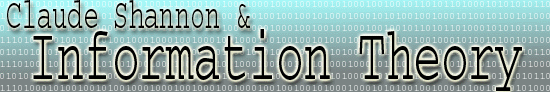Redundancy A simple example of redundancy occurs in the English language. The fact that the letter u always follows the letter q makes the letter combination 'qu' redundant. Instead, the same amount of information could be expressed by a new letter which expresses the same meaning as 'qu.' More formally, redundancy may be expressed in terms of relative entropy. For a given set of symbols, the relative entropy is given by H/Hmax. H is the entropy of the set of states in question with a priori probabilities for each state. Hmax is the maximum entropy for the same number of states. We know that the entropy is at a maximum when all of the states have equal probabilities. For example, in the DNA base problem we have probabilities A = 0.5, T = 0.25, C = 0.125, G = 0.125. The entropy in this particular system is 1.75.  The maximum entropy for a four-state system is 2, when each symbol has probability 0.25. Therefore, the relative entropy in the sample problem is (7/4)/2 = 7/8. The formula for redundancy is R = 1 - H/Hmax (Note R is always less than 1). Therefore, the redundancy in this case is 0.125. In other words, 1/8 of the symbols are redundant. Consider the following message, AAAATTCG with the probabilities  A = 0.5, T = 0.25, C = 0.125, G = 0.125. In contains 8*7/4 = 14 binary digits (1's and 0's) if we encode using the first Fano scheme A = 0, T = 10, C = 110, G = 111. The translation is 00001010110111. However, using the probabilities A = T = C = G = 0.25 and the corresponding Fano code A = 00, T = 01, C = 10, G = 11  consider this message which uses only 7 symbols: AATTCCG. The translation is 00000101101011, which contains 7*2 = 14 binary digits. Since both are Fano codes, each binary digit encodes one bit of information, or the choice between two equally likely possibilities. Therefore, we have encoded 14 binary digits * 1 bit per binary digit = 14 bits of information using 7/8 as many symbols! This is the mathematical meaning of redundancy. Sometimes, redundancy must be added for error-correction on a channel with noise. However, redundancy in the source is invariably bad. Sometimes, it is useful to think of redundancy as "negative entropy", although this is not quite correct. Entropy in the source is good because it conveys more information with less overhead. Redundancy, therefore, is detrimental in the source because it contributes to inefficient codes. However, the entropy caused by channel noise is detrimental (unless the channel is being used as a source, of course). Since redundancy combats entropy, we may add in redundant error-correcting codes to make transmission of the message safer over a noisy channel.